GFG App
Open AppBrowser
Continue

# How to Calculate Spearman Rank Correlation in Excel?

We have noticed a general trend that with an increase in the height of a person, its weight also increases. This happens because there is a positive correlation between height and weight. As one variable increases, the other one also increases, but with this, we only get the quality measure of the data and not quantity, that by how much they are related. To solve this problem, we have a Spearman Rank Correlation coefficient whose value will tell by how two variables are related. In this article, we will learn how to calculate Spearman Rank Correlation Coefficient in excel.

## What is Spearman Rank Correlation Coefficient?

Spearman rank correlation coefficient is a non-parametric measure by which we can have a numerical value of how much two variables are related. Spearman’s rank correlation coefficient works on the ranks and not the data set provided. It would be better to say that Spearman works on ordinal data.

### Range of Spearman Rank Correlation Coefficient

1. If the graph is monotonically increasing, then the spearman coefficient tends to 1.
2. If the graph is monotonically decreasing, then the spearman coefficient tends to -1.
3. If the graph is both increasing and decreasing, the spearman coefficient tends to be 0.
4. A perfect 1 value signifies that data is said to have a perfect positive correlation.
5. A perfect -1 value signifies that data is said to have a perfect negative correlation.
6. A perfect 0 value signifies that data is said to have no relation between two variables.

Hence, the spearman coefficient value lies in the range of [-1, 1], where -1 and 1 are included.

### Advantages of Spearman Rank Correlation Coefficient

As spearman works on ordinal data, so it’s a non-parametric test. The test has no relation to the actual values in the data set. This coefficient test works well with outliers. The correlation value is not distorted if there are significant outliers in the data set.

### The formula for Spearman Rank Correlation Coefficient

A formula has been provided to calculate the Spearman rank coefficient. The formula is:Where,

rs = Spearman Rank Correlation Coefficient,

di = Difference of the rank of the values in the data set,

n = Size of the data set.

Note: The Formula works only if there are no tie ranks in your data set, i.e. there should be only distinct values for each Variable.

For example:

DataSet 1: Variable1: [1, 4, 3, 5], Variable2: [3, 4, 2, 5]

DataSet 2: Variable1: [1, 2, 2, 2], Variable2: [3, 4, 2, 5]

In the above two given data sets, DataSet1 satisfies the condition, and hence the formula could be applied to find spearman coefficient, but DataSet2 do not satisfies the condition, as there are duplicate values in Variable1 of second data set, hence the formula could not be applied to find spearman coefficient.

## How to Calculate Spearman Rank Correlation in Excel?

Before following the procedure to calculate the spearman coefficient, we need to understand two functions in excel, which will be helpful in calculating the coefficient.

### Rank Function

=RANK.AVG(number, ref, order)

The rank specifies the rank of a given number in a dataset; one can also select the order in which rank has to appear. =RANK.AVG() takes three arguments: number, ref, and order.

Argument 1: Number is the first argument in the rank function, which specifies for which number rank has to be estimated.

Argument 2: Reference is the second argument in the rank function. One needs to provide the absolute range of the data set.

Argument 3: Order is the third argument in the rank function. The order can be either ascending(1) or descending(0).

### Correl function

=CORREL(array1, array2)

Similar to the spearman rank correlation coefficient, we also have the Pearson correlation coefficient. Pearson correlation coefficient is a parametric test to calculate the correlation value of two variables. Both the test is nearly the same. Just the difference lies in that spearman works on ranks of the data, and Pearson works on the actual data. The =CORREL() function calculates the Pearson correlation coefficient. This could be very useful in finding the spearman correlation coefficient, which we will talk about in the later stage of the article. =CORREL() function takes two arguments, array1 and array2.

Argument 1: Array1 is the first argument in the correlation function. It takes the entire data set of the variable1.

Argument 2: Array2 is the second argument in the correlation function. It takes the entire data set of the variable2.

## Different methods to find Spearman Coefficient in Excel

There are two different methods by which we can find the Spearman correlation rank coefficient.

### Method 1: Using the Formula

Spearman rank coefficient can be found with the help of a formula, as we have mentioned in the above article, but this formula can only be used if each data set does not contain duplicate values so that the rank of each value is unique. For example, Arushi is an aspiring Chartered Accountant, daily, she used to spend her entire day either studying or playing. For 7 days, she kept track of how many hours does she study and play. On a daily basis, her study hours and playing hours vary. Arushi wants to find whether her playing hours and studying hours are positively or negatively correlated with the help of the Spearman correlation rank coefficient.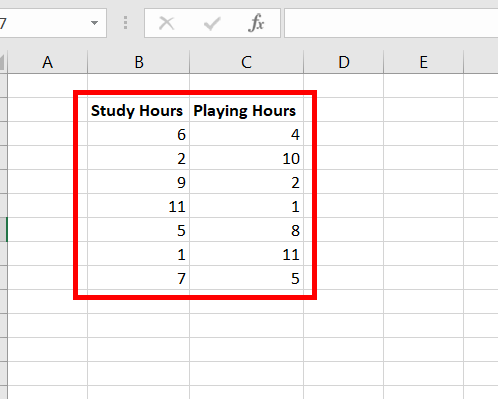### Following are the steps

Step 1: Create a new column name Study Rank. In cell D3, use the formula =RANK.AVG(B3, $B$3:$B$9, 1). This finds the rank of cell B3 for Study Hours. Press Enter.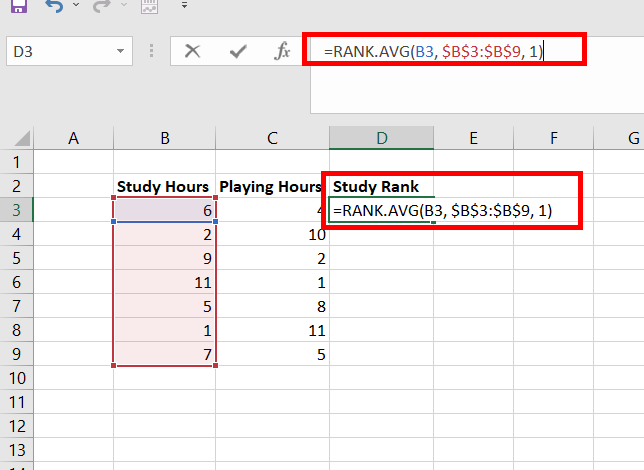Step 2: The number 4 appears in cell D3. This number has ranked 4 in the Study Hours data set.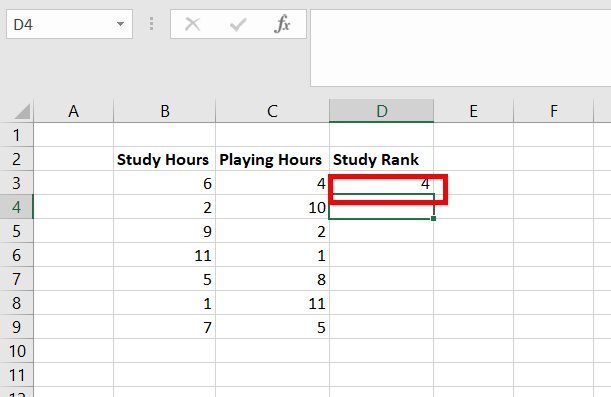Step 3: Copy the same formula of D3 to cells D4:D9.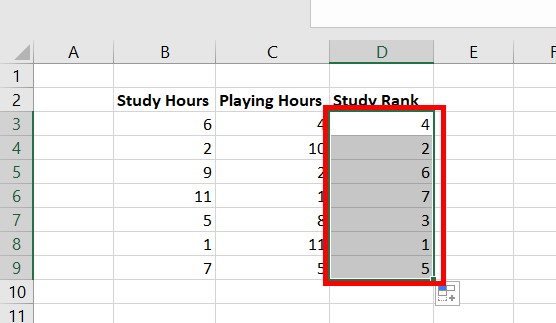Step 4: Create a new column name Play Rank. In cell E3, use the formula =RANK.AVG(C3, $C$3:$C$9, 1). This finds the rank of cell C3 for Play Hours. Press Enter.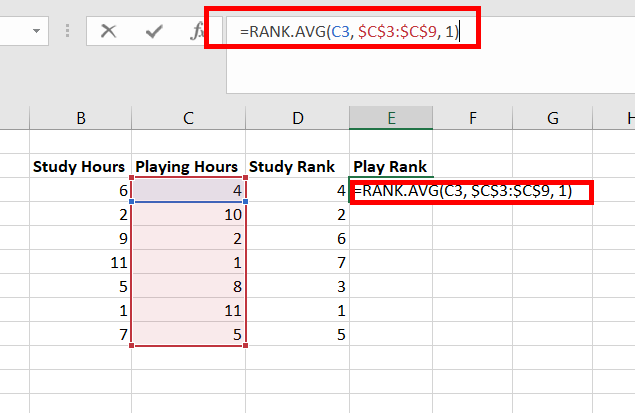Step 5: The number 3 appears in cell E3. This number has ranked 3 in the Play Hours data set.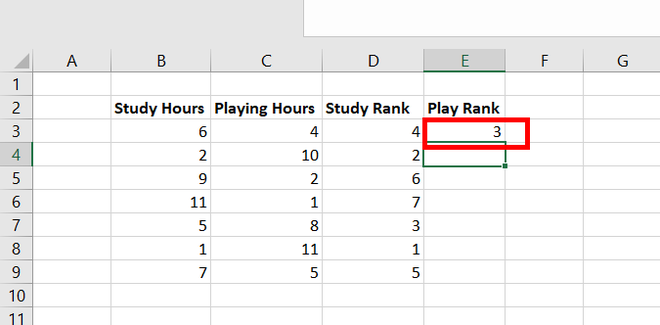Step 6: Copy the same formula of E3 to cells E4:E9.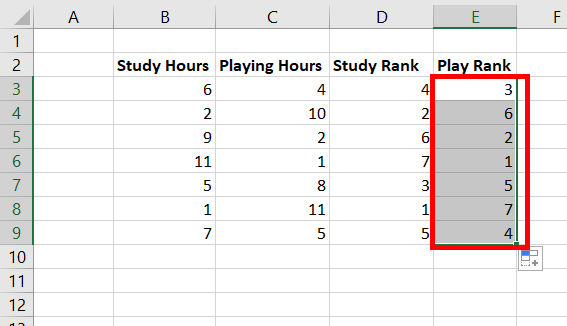Step 7: Create a new column, name, d. In cell F3, use the formula =D3-E3. This calculates the difference in the ranks. Press Enter.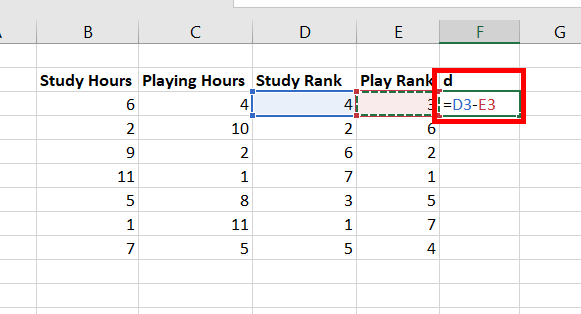Step 8: Copy the same formula of F3 to cells F4:F9.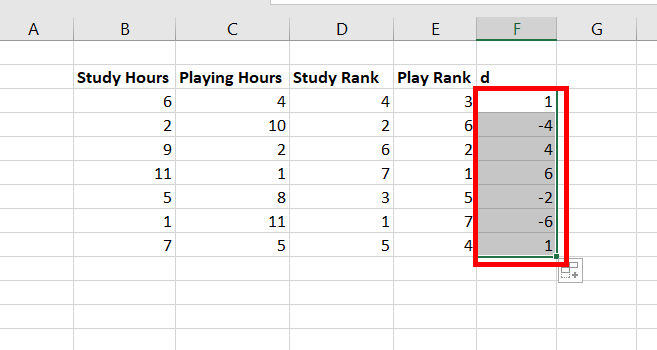Step 9: Create a new column, name, d{square}. In cell G3, use the formula =F3^2. This calculates the square of the difference. Press Enter.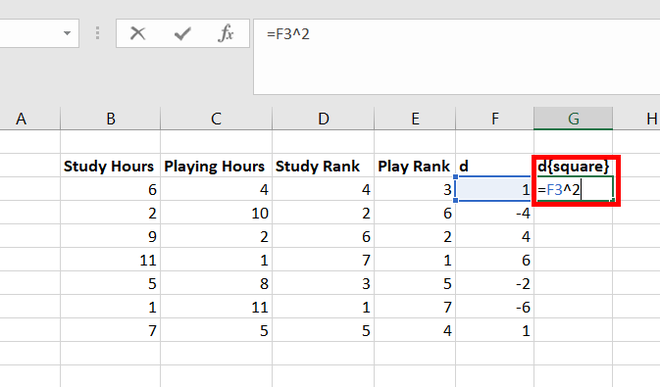Step 10: Copy the same formula of G3 to cells G4:G9.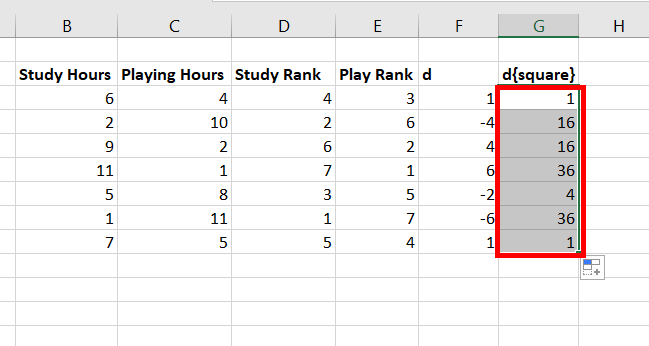Step 11: Use =COUNT(C3:C9) function to calculate the size of the data set. Press Enter.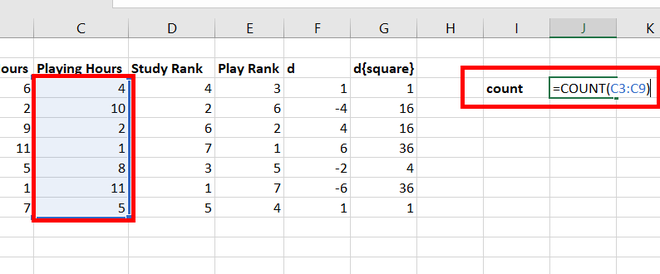Step 12: In the cell, J3, 7 appears, which is the size of the data set.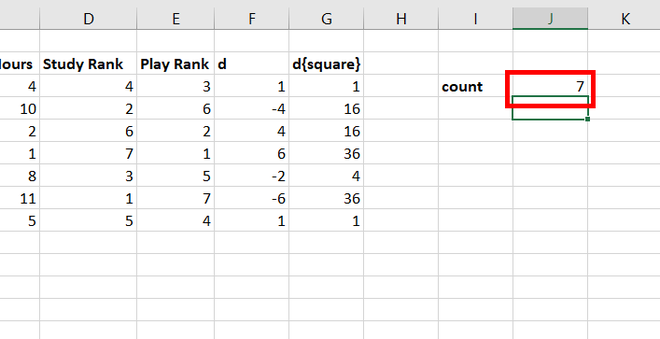Step 13: Use =SUM(G3:G9) function to calculate the sum of the difference between the ranks. Press Enter.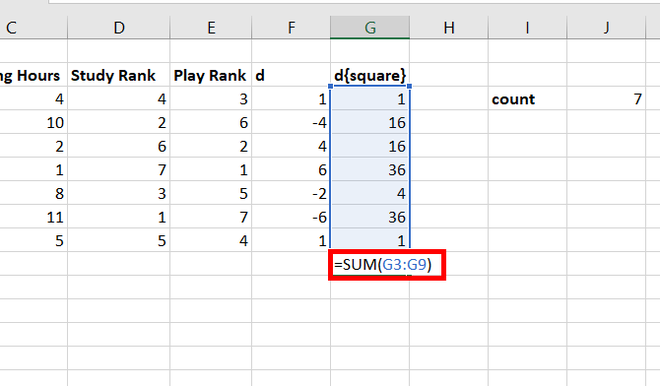Step 14: In the cell, G10, 110 appears.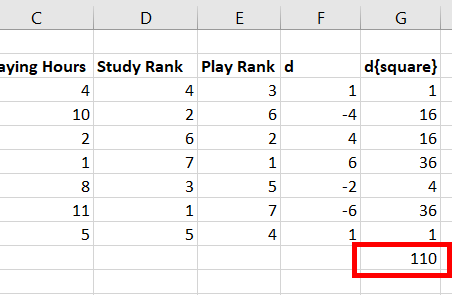Step 15: In cell J5, apply the Spearman formula as mentioned above in the article, i.e., =1-(6*G10/(J3*(J3^2-1))). Press Enter.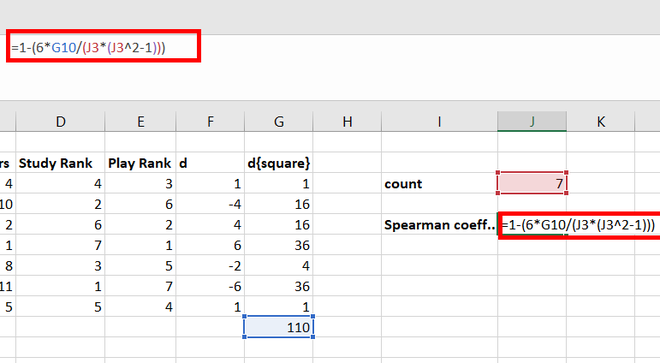Step 16: We get the spearman correlation rank coefficient as -0.96429, which proves that studying hours and playing hours are negatively correlated.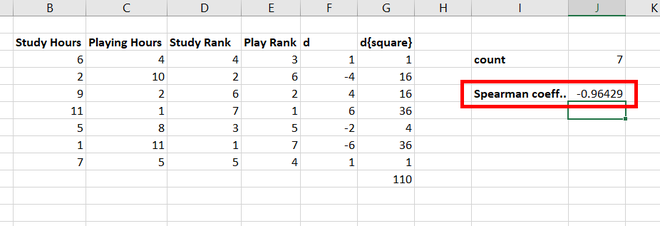### Method 2: Using =CORREL() function

We previously saw that correlated function finds the value of Pearson correlated coefficient by using arguments as data set values. We also know that the spearman coefficient works on the ranks and is a non-parametric test. The correlated function can also be used to find the spearman correlation coefficient by using arguments as data set rank values. For example, Arushi is an aspiring Chartered Accountant, daily she used to spend her entire day either studying or playing. For 7 days, she kept track of how many hours does she study and play. On a daily basis, her study hours and playing hours vary. Arushi wants to find whether her playing hours and studying hours are positively or negatively correlated with the help of the Spearman correlation rank coefficient.### Following are the steps

Step 1: Create a new column, name Study Rank. In cell D3, use the formula =RANK.AVG(B3, $B$3:$B$9, 1). This finds the rank of cell B3 for Study Hours. Press Enter.Step 2: The number 4 appears in cell D3. This number has ranked 4 in the Study Hours data set.Step 3: Copy the same formula of D3 to cells D4:D9.Step 4: Create a new column, name Play Rank. In cell E3, use the formula =RANK.AVG(C3, $C$3:$C$9, 1). This finds the rank of cell C3 for Play Hours. Press Enter.Step 5: The number 3 appears in cell E3. This number has ranked 3 in the Play Hours data set.Step 6: Copy the same formula of E3 to cells E4:E9.Step 7: In cell H4, use =CORREL(D3:D9, E3:E9) function to find the spearman correlation rank coefficient. Press Enter.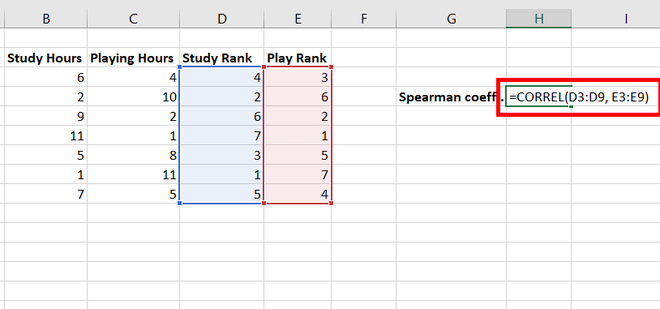Step 8: We get the spearman correlation rank coefficient as -0.96429, which proves that studying hours and playing hours are negatively correlated.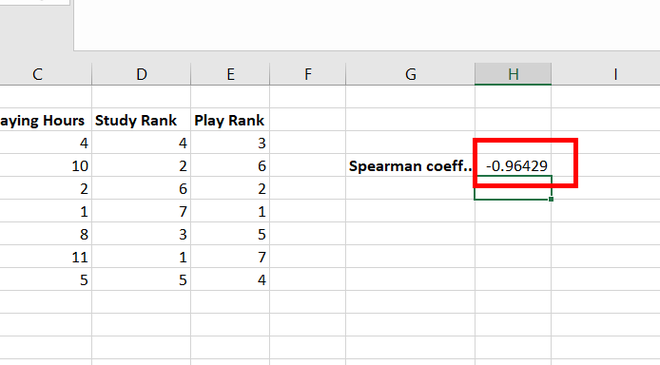My Personal Notes arrow_drop_up